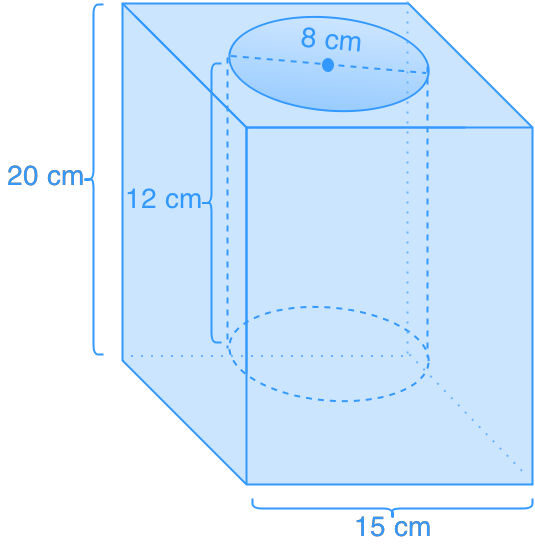Word problems relating volume of prisms and cylinders

Word problems relating volume of prisms and cylinders

We have learned the how to calculate volume of cylinders and prisms. In this section, we will work on word problems which involve both cylinders and prisms.

Lessons

• 1.
A cylindrically-shaped plasticine is 8 cm tall and has a radius of 3 cm. If a kid reshapes this plasticine into a cube, what will be the side length of the cube? Give your answer to two decimal places.

• 2.
A concrete cover of a water cylinder is shown. How much concrete is needed to build this cover?• 3.
What is the volume of a cylinder that can just fit into a cube-shaped box that has a volume of 6000 cm³?